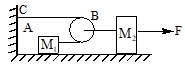In the figure assumes that there is negligible friction between the block and the table. Compute            the tension in the cord connecting  M 1 and the pulley and acceleration of M2. if            M1 = 300 g ,M2=200 g and F = 0.40 N

# In the figure assumes that there is negligible friction between the block and the table. Compute            the tension in the cord connecting  M 1 and the pulley and acceleration of M2. if            M1 = 300 g ,M2=200 g and F = 0.40 N1. A

$\frac{12}{35}\text{N},\text{\hspace{0.17em}}\frac{2}{7}{\text{m/s}}^{\text{2}}$

2. B

$\frac{2}{35}\text{N},\text{\hspace{0.17em}}\frac{12}{7}{\text{m/s}}^{\text{2}}$

3. C

$\frac{7}{2}\text{N},\text{\hspace{0.17em}}\frac{35}{12}{\text{m/s}}^{\text{2}}$

4. D

$\frac{7}{35}\text{N},\text{\hspace{0.17em}}\frac{12}{25}{\text{m/s}}^{\text{2}}$

Register to Get Free Mock Test and Study Material

+91

Verify OTP Code (required)